Divisor Drips and Square Root Waves
by Jeffrey Ventrella

 1. A Pattern-finding Journey 2. Divisor Drips and Reflection Rays 3. The Square Root Spine 4. Fantabulous Parabolas 5. Resonating Waves 6. Relation to the Number Spiral 7. Many Divisors, Many Paths 8. Emergent Patterns 9. A New Appreciation of Number 10. References 11. Acknowledgements

8. Emergent Patterns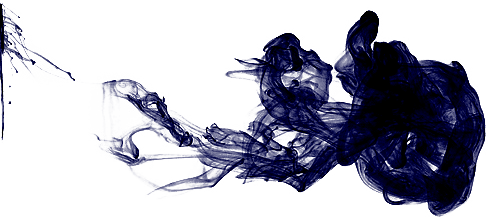Chaotic mixing can be illustrated by stirring paint, repeated folding, or some other iterative operation performed on a simple pattern, whereby it becomes increasingly complex. This kind of process can generate fractal images.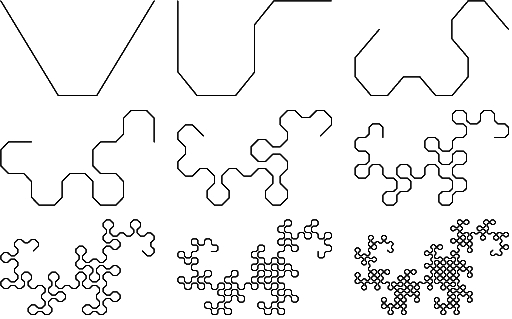In chapter two we considered each row of divisors on the divisor plot as a periodic signal in time along x. The period of each row is equal to y. What happens if the periods are different? Would the same kinds of patterns emerge? The principle of sensitivity to initial conditions applies here: any slight difference in the period of some combination of these signals results in large differences over time (at large x).

To explore this, I made a variation of the divisor plot, ignoring any math and just considering pattern-formation from adjusting the periods.

HERE is an animation of the process (it's a Java Applet) - check it out!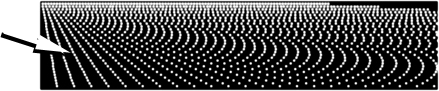The image below shows this transformation.There are eight panels: each showing a stage of the transformation. The top panel shows a rectangular array of dots. In the second panel, the rows of dots begin to expand rightward - but at differing rates: the top row doesn't expand at all and the bottom row expands the most. The difference in expansion among consecutive rows is linear. The last panel at the bottom shows the final result: the divisor plot.

Three distinct features emerge throughout this transformation: (1) diagonal linear features at the bottom-left (corresponding to the first few zero reflection rays), (2) small linear features at the top that come and go throughout the transformation (corresponding to divisor drips and reflection rays), and (3) a series of parabolas near the middle. The series corresponding to the square root boundary is the most distinct and robust throughout the transformation, becoming quite distinct by the third panel.

The robustness of this parabola series got me curious. So I made some variations on this transformation using non-linear shifts among the rows. These also produced parabola series that seemed equivalent (or at least analogous) to the square root boundary. Why are parabolas so common and robust? Answering this question purely from a pattern formation point of view might help to give us fresh eyes to look at the number-theoretical questions about parabolas.

Do Divisor Drips and Parabolas Avoid Each Other?
Parabolas and divisor drips (with their corresponding reflection rays) are perhaps the most visibly-striking features of the divisor plot. Is there some relation? We have already seen that there are many divisor drips - corresponding to spikes in the divisor function, where highly-composite numbers lie. I have a hypothesis: these two different features cannot be found near each other: in fact, they avoid each other! Think about this: divisor drips tend to be densest near the top of the divisor plot. Do any divisor drips reach down and traverse square root parabolas? After exploring parabolas in depth, we would not expect anything of the kind. Why, who would even think of interfering with the majestic flow of parabola waves pointing towards infinity!

But it may not be as simple as I have described it. Divisor drips have a clear origin (the top of the divisor plot, where density is 1), but their density tapers off as the drips fall downward. Where a divisor drip ends is perhaps just a matter of opinion. I leave it to you to either prove me right (there is something fundamentally incompatible about divisor drips and parabolas), or to prove me wrong (divisor drips and parabolas can live in harmony, and they have a secret collaboration that is not readily seen upon first glance).

The Divisor Plot is Self-Similar
When visualizing the structure of the number line, it is easy to find self-similar, scaling patterns, as Wolfram and others have shown, such as with the patterning in the binary expression of the number line, in which 0 is light gray and 1 is black.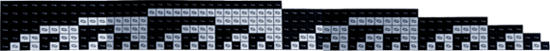The family tree of composite numbers is self-similar. Every composite number is made of multiple copies of parts. Most composite numbers have parts made of parts made of parts, and so on. One way to appreciate the idea of fractal self-similarity is to create a fractal using pen and paper, and photocopy machine. Or else you could use a graphics application like Photoshop, with simple processes like cut-and-paste. The same works for the divisor plot.

You do not have to be a programmer to generate nice images of the divisor plot. Take a look at the illustration below, which shows such a process. You will notice that at each stage, copies of copies of copies are made.Each time a new row of numbers is added at the bottom, it is offset to the right by one unit, building the familiar diagonal zero reflection ray at the left. As you can see, the divisor plot is emerging before your very eyes.

You may have noticed something else in this accumulating pattern: the last column at the right of the pattern is always full of divisors, i.e., it is a solid divisor drip. Take a look at the next few steps: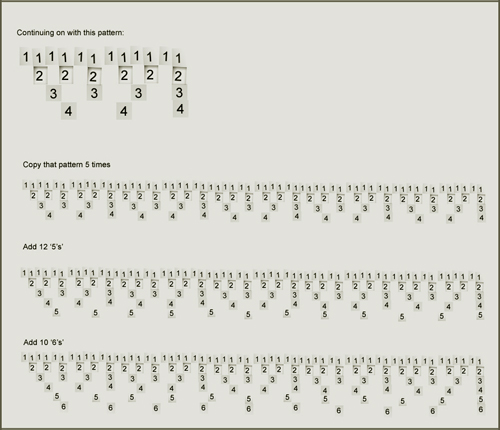The zero reflection ray at the left of the pattern is increasing steadily, and a continuous divisor drip at the right is increasing as well. Nice and tidy! What a comfortable feeling to know that the left and right sides of this pattern provide orderly boundaries, keeping all that chaos inside.

Did you notice something else? The entire pattern is symmetrical. If you remove the divisor drip at the right, then you will find that the remaining left and right halves of the pattern become perfect mirror images of each other. We saw this earlier in the chapter on divisor drips while exploring the repetition of factorial patterns every n! number.

Something curious occurred while I was working out this construction algorithm: in order to insure a solid divisor drip at the right at each step, the exact number of numerals to add at the bottom, and the exact number of copies to make had to be precise. But, as the table below shows, the series of parameters I chose is not orderly (or not apparently so). This is not what I expected at first. Check it out.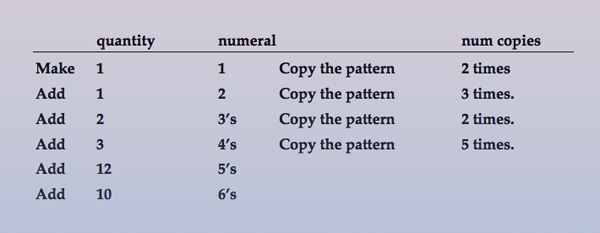How do you explain the erratic series of numbers in the left and right columns? I will give you a hint: there should be another column of numbers in the table: at the very right I should have included a column of numbers listing the value of x, representing the number line, visualized as the length of the pattern at each step of the process.

Now, can you guess what the next step is in this process? I'll tell you the answer: "Copy that pattern 7 times." And there is another hint. It seems that the number of copies must always be a prime number!

Want to See More Structure? Visit a Billion...and Beyond
I would not recommend using Photoshop or a photocopy machine to construct the divisor plot as I have just demonstrated if you want to see the patterns in really large numbers. For that, you are advised to do a bit of programming, especially if you are interested in numbers whose names end in "illion".

Let's peer into the large numbers. Below is an image of a large area of the divisor plot at two billion. Notice that the parabolas appear flattened-out, and the spaces on either side of the parabola vertices are empty, as if they were bullets passing through a dusty cloud. Also, notice that there are many, many parabolas scattered throughout. Some of them seem to have appendages, puffs, and pseudopods extending out from them. Perhaps I am hallucinating. Perhaps I am exercising too much visual poetic license. On the other hand, this visual poetry is what motivates me (and I think you too :) to keep looking deeper.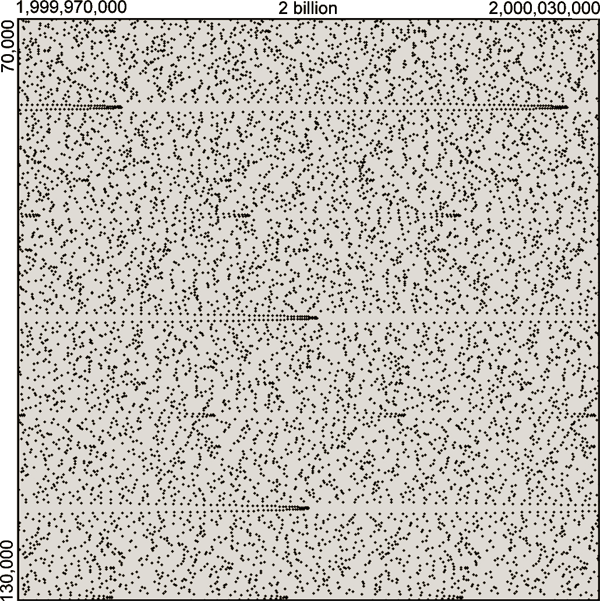It seems that as we travel to higher numbers, these patterns exhibit more kinds of periodic structure. Perhaps the complexity is related to the hierarchical nature of large composite numbers - which are the products of smaller numbers, some of which themselves are the products of smaller numbers, and so-on - terminating in prime factorization.

Let's Visit One Quintillion: 1,000,000,000,000,000,000
One billion times one billion is one quintillion, or 1018: one followed by eighteen zeros. Below is a view of the divisor plot ranging from one quintillion - 20,000 to one quintillion + 20,000, among the first 20,000 divisors. Not much to see here among such small divisors. In fact, the only thing this really shows is how the average density of the divisor plot ranges from 1 to 0, nonlinearly from top to bottom.Let's scan downward and take a look at the square root boundary. Here we see the square root parabola whose vertex is located at y = one billion.

This illustration shows six consecutive magnifications outward, starting with a window of 100x100 and zooming out to a window of 10,000,000.Notice how much empty space there is around this parabola's vertex, compared to the distance between consecutive divisors along the curve. At lower-left, we begin to detect a few divisors coming into view. As we zoom out farther, the square root parabola appears thinner, almost needle-like. This vast empty space around an otherwise dense parabola is something we have seen before. But it appears to become more pronounced in the higher numbers. What accounts for this property of the square root boundary? I invite you to come up with reasons (because I have no clue!)

Is one quintillion too small for you? Below is an image showing the square root of nine quintillion, in a 20,000,000 x 20,000,000 window.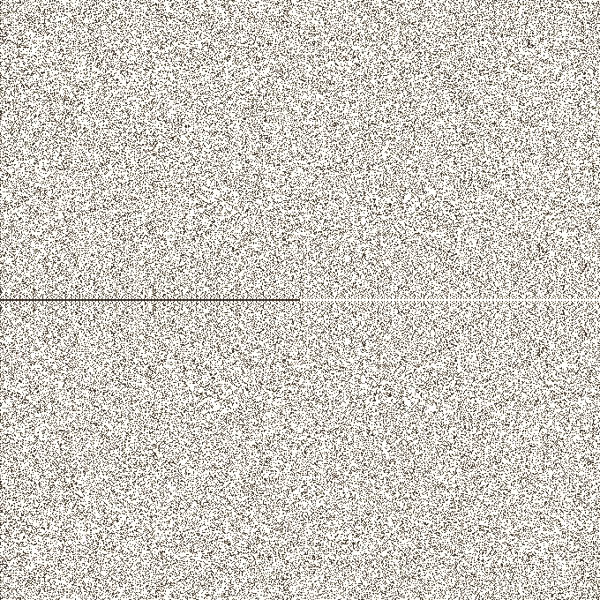This image shows a lot of symmetry about the square root boundary. There may be some kinds of symmetry in other parts of this region, but we can't easily see it.

There is something else that is quite novel upon exploring these very large numbers. Let's set our gaze back to one quintillion now. If we scan down along the divisors to y = one million, we see a curious object, shown below.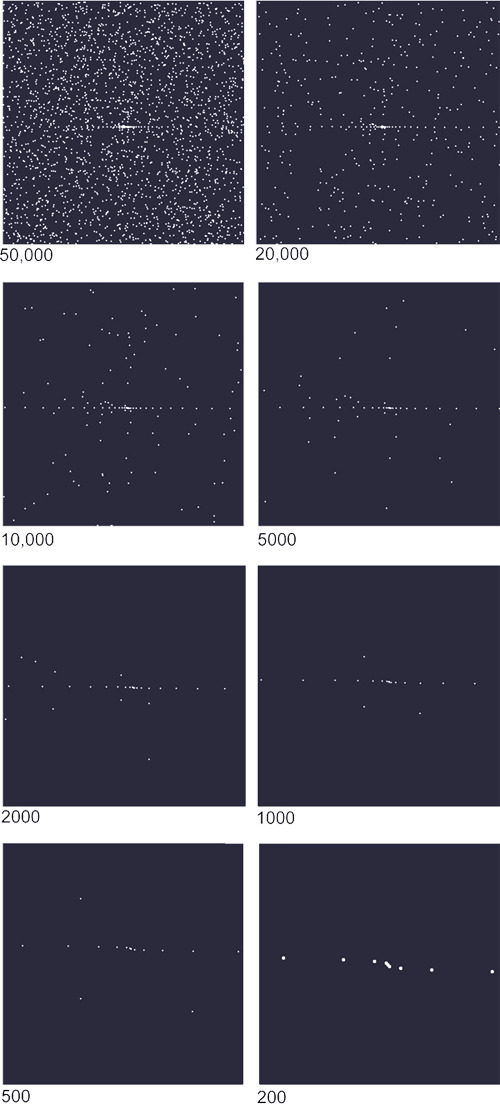I have rendered the divisors in this object as white dots against black because it's fun to pretend like we are peering through a telescope at a distant galaxy. This series of images zooms in to this location, starting at a 50,000 x 50,000 window and ending at a window of 200 x 200.

Have we just discovered a new structure? Is this the result of numerical error in my code? This note of caution is based on the account of Benoit Mandelbrot's first sighting of the Mandelbrot Set, in which he thought there may have been an error, causing the fractal-like artifacts, which turned out to be actual features of the Mandelbrot Set.

In the chapter on parabolas I described the phenomenon of parabola series occurring along curves defined by y = (square root of x) times n, and y = (square root of x) divided by n. These are the same as the product curves discovered by Rob Sacks. When x = one quintillion, then one million = (square root of x) / 1000. Based on this fact, I decided to explore other values of n. Below is an illustration showing features with n of 200 (left), 500 (middle), and 2000 (right), each in a window of 5000 x 5000.I then set x to 10243, which is 1,073,741,824, and looked at y = (square root of x) / n, with n of 32. A similar pattern is shown below.This little 3-divisor diagonal motif seems to pop up often, like a tiny descending flute melody. I discovered that this motif is always found at cube roots. I checked the small cube roots from 2 to 7, to make sure. The images below show the first few instances of this motif.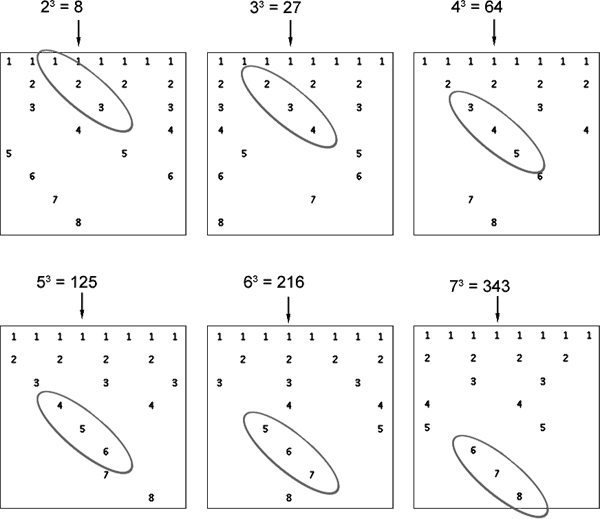This motif continues on to the larger cubed numbers, as shown in the example below of 977 cubed. I then realized that the cube root of one quintillion is one million - I hadn't realized this earlier when we were looking at this divisor location. (In these examples, the y axis is scaled to make the numbers easier to read.)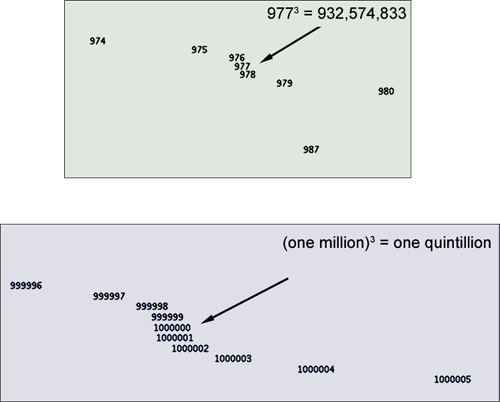If all cube root divisors do indeed have this motif, then we have the following conjecture: all cube numbers greater than 23 have composite numbers as their immediate neighbors. No cube number has a prime neighbor.

One such cubed number I tested was 323 = 32768. Its motif is shown below. Notice that this motif is reflected about the divisor drip D32736.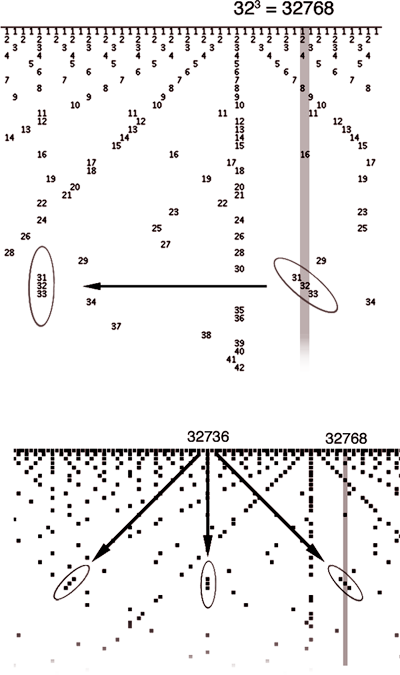Beasts on the Horizon
I would not describe these flute motifs as "parabolas". But, might they be morphologically related? Distant cousins? My big question is this: do more novel structures like this lie at astronomically large regions of the number line? Do the kinds of structures continue to increase as well? Do these cube root motifs grow more appendages among the larger numbers? And what about other power numbers? Might those pseudopods we saw earlier be the embryos of something magnificent that awaits our eyes when we can explore numbers like 101000?

These motifs are perhaps the tip of a whole new iceberg waiting to be melted. Would you like to know the answers to these questions? Well, the reason I have no more answers is very simple: if I were to continue down this line of inquiry, and then address all the new questions that would result from consequent discoveries, I would NEVER FINISH THIS BOOK. The temptation to keep exploring, graphing, looking, writing, and conjecturing is enormous. And while the joy of discovery is a major motivation for this book, I need to stop at some point! (Happily, if you are reading this book now, I have somehow managed to finish it and get it into your hands).

We have accumulated many unanswered questions. And so, I would say that this journey has been a great success.

Randomness vs. Chaos
The digits in Pi are indistinguishable from a random sequence. If you search long and hard enough, you will find any sequence of digits in Pi, including your own phone number. Since the divisors in the number line are not random, I would not expect any possible pattern to be lying out there, no matter how far you look, such as the likeness of Elvis. But it does seem that there is more complexity and more kinds of complexity as I travel to higher numbers. So, I am very curious as to what may be found if I were to beef up my computer program to use gigantic numbers. This is certainly on my to-do list.

Next chapter: 9. A New Appreciation of Number